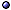Constraint Equations in General Relativity

In General > s.a. canonical formulation [constraint algebra]; initial-value formulation; ADM and connection formulation.
* Idea: They are the Einstein equation with one or more indices projected arthogonally to a spacelike hypersurface or, in differential geometry terms, just the Gauss-Codazzi equations with the Ricci tensor substituted for in terms of the matter stress-energy.
* In terms of first and second fundamental form: For real, Lorentzian gravity

Gab qma nb = Da KamDm K = 8πG Tab qma nb = 8πG jm

2 Gab na nb = 3RKab Kab + K2 = 16πG Tab na nb = 16πG ρ ,

where N:= [−(∇a t) (∇a t)]−1/2 is the lapse, na:= −Na t is the unit normal to Σ, and K:= Kaa = qab Kab.
* For complex / euclidean general relativity: Flip the signs of the two KK terms in the second constraint equation.
@ General references: Moncrief PRD(72) [redundancy], & Teitelboim PRD(72) [Hamiltonian and diffeomorphism]; Dittrich CQG(06)gq/05 [diff-invariant Hamiltonian constraints]; Balasin & Wieland a0912 [simple Hamiltonian constraint for specific value of the Barbero-Immirzi parameter]; Mars GRG(13)-a1303 [for general hypersurfaces, and applications to shells]; Rácz CQG(16)-a1508 [as evolutionary systems].
@ On closed / compact manifolds: Isenberg CQG(95) [constant mean curvature], & Moncrief CQG(96) [non-constant mean curvature]; Choquet-Bruhat CQG(04)gq/03-in [compact nΣ]; Maxwell JHDE(05)gq [rough/low-regularity]; Holst et al PRL(08) [far-from-constant mean curvature], CMP(09)-a0712 [without near-CMC assumption]; Dilts CQG(14)-a1310 [with boundary]; Canepa et al AHP-a2010 [with null boundary].

Variables and Solution Methods
* Lichnerowicz-York solution method: A conformal technique initiated by Lichnerowicz and perfected by York, the only efficient and robust method of generating consistent initial data; In the spatially compact case, the complete scheme consists of the ADM Hamiltonian and momentum constraints, the ADM Euler-Lagrange equations, York's constant-mean-curvature (CMC) condition, and a lapse-fixing equation (LFE) that ensures propagation of the CMC condition by the Euler-Lagrange equations; The variables are a conformal factor ψ, a spatial metric gij, a symmetric tensor Aij and a scalar τ, in terms of which the physical metric and extrinsic curvature are

hij = ψ4 gij,     Kij = ψ−2 Aij + $$1\over3$$ψ4 gij τ ;

The Hamiltonian constraint is rewritten as the Lichnerowicz-York equation for the conformal factor ψ of the physical metric ψ4 gij, given an initial unphysical 3-metric gij; The CMC condition and LFE introduce a distinguished foliation (definition of simultaneity) on spacetime, and separate scaling laws for the canonical momenta and their trace are used.
@ General references: Isenberg & Marsden JGP(84) [York map]; Maxwell CMP(05)gq/03 [constant mean curvature conformal method]; Anderson et al CQG(05)gq/04 [physical degrees of freedom]; Pfeiffer et al PRD(05) [stationary + gravitational wave]; O'Murchadha APPB(05)gq [uses of Lichnerowicz-York equation]; Tiemblo & Tresguerres GRG(06)gq/05 [and Poincaré gauge theory, single condition]; Martín et al IJGMP(09)-a0709 [framework for solutions]; Maxwell a0804 [freely-specified mean curvature]; Corvino & Pollack a1102-fs; Maxwell CQG(14) [conformal method and conformal thin-sandwich method]; Tafel GRG(15)-a1405 [(2+1)-decomposition approach]; Cang AHP(15)-a1405 [application of fixed-point theorems]; Alves a1603-wd [Hamiltonian constraint, reduction to a first-order equation]; Anderson ATMP-a1812 [Lichnerowicz-Choquet-Bruhat-York conformal method, in the far from CMC-regime]; Canepa et al a2001 [tetrad variables].
@ And conformal structure: Beig & Ó Murchadha CMP(96)gq/94 [and spatial conformal Killing vectors]; Szabados CQG(02)gq/01 [Hamiltonian and Chern-Simons functional]; Butscher CMP(07)gq/02 [conformal constraint equations]; Pfeiffer gq/04-proc [conformal method]; Pfeiffer & York PRL(05), Walsh CQG(07) [non-uniqueness of solutions]; Gourgoulhon JPCS(07)-a0704 [and 3+1 numerical initial data]; Holst et al a0708; Nguyen a1507 [non-existence and non-uniqueness results].
@ Black holes: Brandt & Brügmann PRL(97) [multi-black-hole]; Loustó & Price PRD(97), PRD(98) [data for binary collisions]; Baker & Puzio PRD(99)gq/98 [axisymmetric]; Dain et al PRD(05)gq/04 [multi-black-hole]; Smith GRG(09) [horizon with prescribed geometry]; Baumgarte PRD(12)-a1202.
@ Binary black holes: Baumgarte PRD(00)gq; Marronetti et al PRD(00)gq, & Matzner PRL(00)gq [arbitrary P, L]; Dain PRD(01)gq/00 [2 Kerr, head-on].
@ Asymptotically hyperbolic: Isenberg & Park CQG(97)gq/96; Sakovich CQG(10)-a0910 [Einstein-scalar, constant mean curvature solutions]; Gicquaud & Sakovich CMP(12)-a1012 [non-constant mean curvature].
@ Rough / low-regularity solutions: Maxwell gq/04; Behzadan & Holst a1504 [asymptotically flat, non-CMC].
@ Other solutions: Maxwell CMP(05)gq/03 [with apparent horizon boundaries]; Choquet-Bruhat et al gq/05, CQG(07)gq/06, gq/06 [Einstein-scalar]; Korzyński PRD(06)gq [on dynamical horizons]; Huang CQG(10) [with prescribed asymptotics]; Tafel & Jóźwikowski CQG(14)-a1312; > s.a. models in numerical relativity.

References > s.a. numerical relativity; canonical quantum gravity.
@ Reviews: Bartnik & Isenberg gq/04-proc; Carlotto LRR(21).
@ Gluing solutions: Isenberg et al CMP(02)gq/01 [and wormholes], AHP(03)gq/02; Isenberg gq/02-GR16; Chruściel et al CMP(05)gq/04, PRL(04)gq [more general]; Isenberg et al ATMP(05)gq [with matter]; Chruściel et al CMP(11)-a1004 [N-body initial-data sets]; > s.a. solution methods.
@ Space of solutions: Ó Murchadha CQG(87) [ADM energy as Morse function]; Chruściel & Delay JGP(04)gq/03 [manifold structure]; Dain gq/04-proc [black holes as boundaries]; Rai & Saraykar a1605 [coupled scalar fields, Hilbert space structure]; Holst et al a1711 [drift method, rev and applications].
@ In other gravity theories: Jacobson CQG(11)-a1108 [generally-covariant theories with tensor matter fields].
@ Constraint propagation: Frittelli PRD(97) [and numerical evolution]; York gq/98 [and canonical formalism]; York a1512-wd [causal propagation].
@ Related topics: Kuchař & Romano PRD(95)gq [sets that generate true Lie algebras]; Frauendiener & Vogel CQG(05)gq/04 [instability of constraint surface]; Gambini & Pullin GRG(05)gq-GRF [getting rid of constraints in discretization]; Corvino & Schoen JDG(06) [vacuum, asymptotics]; Bojowald et al PRD(06)gq [effective constraints from lqg]; Szabados CQG(08)-a0711 [Hamiltonian constraint for Einstein-Yang-Mills theory as Poisson bracket]; Ita a0904v5 [diffeomorphisms and Gauss' law]; Holst & Kungurtsev PRD(11)-a1107 [bifurcation analysis of conformal formulations].Various theories: see formulations of general relativity; linearized general relativity; modified gravity.